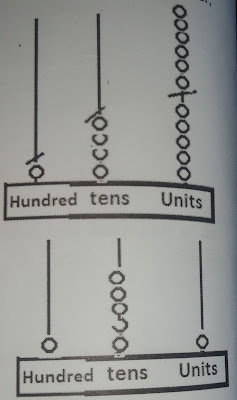## Lesson Plan of Addition up to 100

Lesson Plan of Addition up to 100 Subject Mathematics Grade III Students` Learning Outcomes Add numbers up to 100 using …

## Lesson Plan of Addition (4-digits with carrying)

Lesson Plan of Addition (4-digits with carrying) Subject Mathematics Grade III Students` Learning Outcomes Add numbers up to 4-digits (with …

## ADDITION AND SUBTRACT UNITS OF TIME

LESSON PLANNING OF ADDITION AND SUBTRACT UNITS OF TIME WITH CARRYING/BORROWING Subject Mathematics Grad 5th Students` Learning Out coming …

LESSON PLANNING OF ADDITION OF 3-DIGIT NUMBERS WITH CARRY OF TENS AND HUNDREDS Subject Mathematics Grade 2nd Students` Learning …

LESSON PLANNING OF ADDITION WORD PROBLEM Subject Mathematics Grade 2nd Students` Learning Outcomes Add 3-digit numbers and 3-digit numbers without …

LESSON PLANNING FOR ADDITION OF_DIGIT NUMBERS WITHOUT CARRY Subject Mathematics Grade 2nd Students` Learning Outcomes Add 3-digit numbers and …

## Lesson Plan of Addition of Two Digits with Carry

LESSON PLANNING FOR ADDITION OF TWO DIGITS NUMBERS WITH CARRY Subject Mathematics Grade 2nd Students` Learning Outcomes Add 2-digit …

Lesson Planning of Number Operation (Addition, Subtraction) Subject Mathematics Grade II Students` Learning Outcomes Solve real life problems (using …

## Number Operations (Addition with carrying)

Lesson Planning of Number Operations (Addition of 3-digit numbers with carrying)

Subject Mathematics

Students` Learning Outcomes

• Add 3-digit numbers and ones with carrying of tens and hundreds.

Information for Teachers

• Question should be written vertically and column headings (units, tens, and hundreds) should be mentioned. Sign of addition + shouldn’t be written under any column heading. There must be space between column headings and numbers to write carry.• While teaching the lesson, the teacher should also consult textbook at all steps wherein and whenever it is required.

H    T    U

4     5    6

+ 7    4    3

___________

Material / Resources

Writing board, chalk/marker, duster, beads, counting frame, clips, paper strips, dices (you can make your own dices using cardboard from empty cartons. Make them bigger than the usual dice, like a 3 x 3 inch dice with numbers written on each side in bold writing)

Introduction

• Write an addition problem on the board (3-digit with 2 or 3-digit numbers without carrying) on each strip e.g. Mala got 200 rupees as Eidy from her brother and Rs. 450 Eidy from her father. How much does she have altogether?
• Ask students to solve assigned questions on their notebooks.

Development

 Activity 1 Write an addition question (3-digit with 1-digit on writing board (e.g. 145 + 6) and provide a counting frame to students in group. Ask students to represent 145 on counting frame by putting five beads, four beads and one bead in unit, tens and hundred string respectively.Ask them to clip each string and then represent second added by putting six beads in unit string. Ask them to remove clip in unit string, count and when reach at ten remove them from unit string and exchange with one bead at tens string. Write carry under ten`s place on writing board. Count beads in unit string and write beads 1 under the unit on notebooks. Combine beads in tens place, count and write number of beads under the column of tens on writing board. Then count number of beads in the string of hundred and write under the column of hundred on writing board. Give a few more questions of the same level of difficulty level e.g. 196+5. Ask students to repeat step 2 to 8 and find the answer of question.

 Activity 2 Distribute strips containing an addition question (3-digits with 1-digits numbers) e.g. 167+4, 435+7, 796+7, and 193+8 in groups. (you can also write the questions on the board for each group) Ask students to solve the question in groups. After solving question ask students to share with other groups how did they solve given question.

 Activity 3 Give three dices to students in group and ask them to roll them. Ask them to use it all three drawn digits to form a three digit biggest possible number. Discuss with them how that can be done: by placing the largest of the 3 numbers on the place value hundred. Ask them to add 9 to their formed number and share sum with other groups. Group having greatest sum will be the winner group.

Sum up / Conclusion

• Discuss with students what will happen when the sum of two digits becomes more than nine.
• Demonstrate with material that as soon as a number become more than 9 we replace ten with one in the next column heading and this process continue for next place.

Assessment

• Give counting frame to students along with some questions to solve e.g. 445+5, 765+6, 794+9, 699+8, etc.
• Teacher is also required to involve the students in solving the problems given in the exercise at the end of unit/chapter.

• Assign some addition questions (3-digits with 1-digits numbers with carry) to solve as homework.
• Sample Questions:

H   T    U

4    5    6

+            7

H   T    U

9    8   7

+           9